# Catch up on the latest AI articles# [Materials Informatics] CGCNN-Transfer Learning Model For Data Deficiency Of Physical Property Values

3 main points
✔️ Crystal Graph Convolutional neural network (CGCNN) is proposed for transition learning (TL-CGCNN)
✔️ In Crystal Graph descriptor, only the crystal structure of a material is used as an explanatory variable
✔️ Pre-training with big data of easily obtainable physical properties enables highly accurate prediction of other physical properties that are difficult to obtain.

written by Joohwi LeeRyoji Asahi
(Submitted on 20 Jul 2020 (v1), last revised 29 Jan 2021 (this version, v4))
Comments: Published in Comp. Mater. Sci

Subjects: Materials Science (cond-mat.mtrl-sci); Computational Physics (physics.comp-ph)code：The images used in this article are from the paper or created based on it.

## How to deal with the problem of poor prediction performance due to insufficient data...

Unlike fields such as computer vision and natural language processing, the number of accumulated data in the field of MI (Materials Informatics) tends to be small, and there is a problem that machine learning does not provide sufficient prediction accuracy. This is because the amount of data obtained by experiments is limited, and even simulations using computational science often require huge costs to calculate.

One of the solutions is transfer learning, which has recently attracted a lot of attention in the field of MI. The idea is that a model that has been pre-trained on a property value for which big data exists can be used to predict another property value.

## descriptor selection

Another important challenge in materials research is to develop versatile descriptors of materials that can predict various target variables (property values). Since the physical properties of material strongly depend on its crystal structure and constituent elements, many descriptors based on these structural properties have been developed. Examples are the Coulom matrix, SOAP (Smooth Overlap of Atomic Positions), and R3DVS (Reciprocal 3D voxel space).

Recently, CGCNN (Crystal Graph Convolutional neural network) has been proposed by Xie and Grossman, which requires only the crystal structure of material for classification and prediction tasks. The only information required for the classification and prediction task is the crystal structure of the material, and the crystal graph structure is created from the crystal structure, and the deep neural network predicts the target variable (property value) based on it.

When using a descriptor to perform transfer learning, it is important to be able to accurately predict physical properties that have a low correlation with the physical properties used in the pre-training. If the descriptor does not capture the characteristics of the material structure from the ground up, it will not be possible to perform transfer learning for various properties.

In this study, we investigate the performance of a model that combines CGCNN and transition learning (TL).

## model building

### CGCNN

CGCNN consists of a part to create graph structure from the crystal structure and a part of deep CNN which consists of embedding layer, convolutional layer, pooling layer, and all joining layers.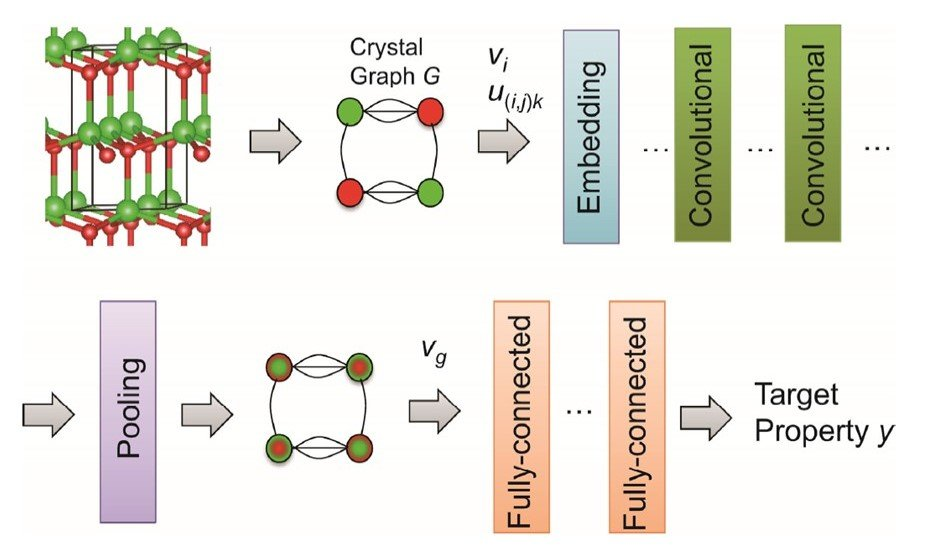A crystal graph G is represented as a discrete descriptor of groups of atoms, atomic numbers, and distances between atoms expressed as binary numbers. Nodes represent atoms and edges represent chemical bonds, consisting of a set of atoms in the crystal structure, indirect bonds, atomic properties, and bond properties. This discrete descriptor is then transformed into a continuous descriptor in the embedding layer. The continuous descriptors are then input to the convolution layer.

In the i+1th convolutional layer, the atomic property vector vi is represented as follows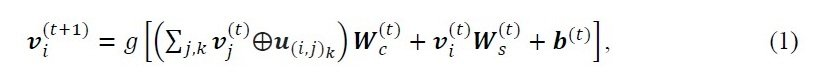u(i,j)k: bond property vector (the kth bond between the i-th and j-th atoms) Wc: the weight matrix of the convolution, and Ws: weight matrix of self, b: bias of the t-th layer, g: soft plus function, a symbol between v and u: concatenation of v and u

However, the above equation has a problem that it is difficult to recognize the interaction between individual atoms because the atom and bond vectors of all neighboring atoms in the crystal structure share a weight matrix. Therefore, we apply the standard edge-gating technique. To do so, we use a vector z(i,j)k that summarizes the features between neighboring atoms to represent the atomic property vector vi as follows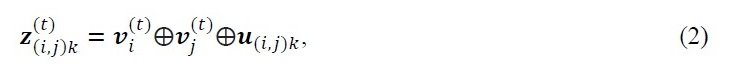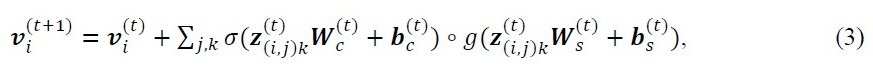σ: sigmoid function, ○: element-wise multiplication of σ

Xie and Grossman report better prediction performance using this method. vi is then input to the pooling layer. In this case, average pooling is used to obtain the crystal feature vector vg.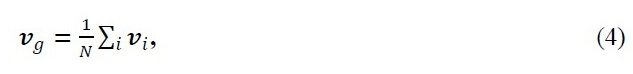N is the number of atoms in the crystal graph

Finally, vg is input to the full coupling layer. Here, the system is trained to calculate the objective variable (property value) from vg using a nonlinear function.

### TL-CGCNN

In this study, fine-tuning is employed as a method to incorporate parameters optimized by pre-learning.

Incidentally, tanh standardization was performed to align the scales of the objective variable (property values) between the pre-training task and the objective task.

The crystal structures of the materials and the corresponding physical properties such as bandgap energy (Eg) and formation energy (ΔEf) were prepared from the Materials Project Database (MPD), an ab initio database. energy (ΔEf) The TL-CGCNN models were pre-trained with data from the

(If you are interested in learning more about the details of the study, you can refer to the paper.)

## Results and Discussion

### Comparison between CGCNN and TL-CGCNN

In the following, the model is shown as follows.

Ex.) 500-NM-Eg: The target variable is the bandgap energy (Eg), the number of training data is 500, and only data of nonmetallic material (NM) are used.

Most of the materials we work with are bulk (not nanoparticles, thin films, etc.) inorganic materials.

The following figure shows a scatter plot between the formation energy (ΔEf) and the bandgap (Eg) for all 118286 materials in the database. The correlation coefficient (rp) in the linear regression is -0.49. There is no strong correlation between the properties.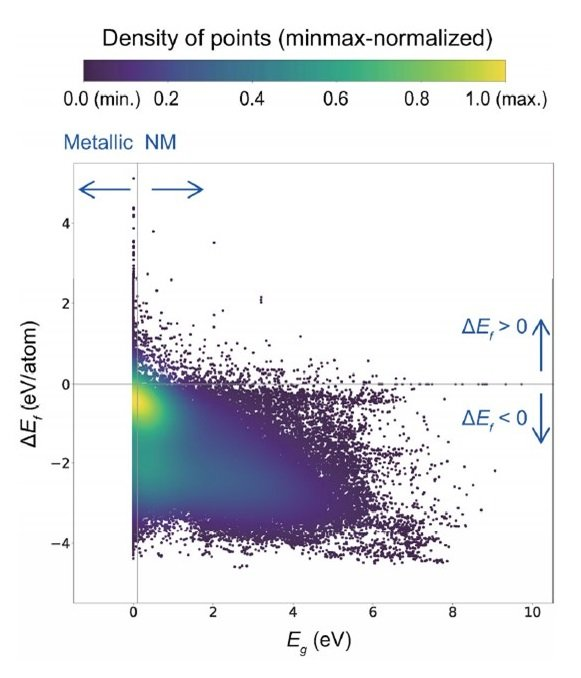The color scale represents the relative density obtained by Gaussian kernel density estimation.

The following figure shows the correlation between dataset size and prediction error for the respective prediction tasks of Eg and ΔEf in CGCNN.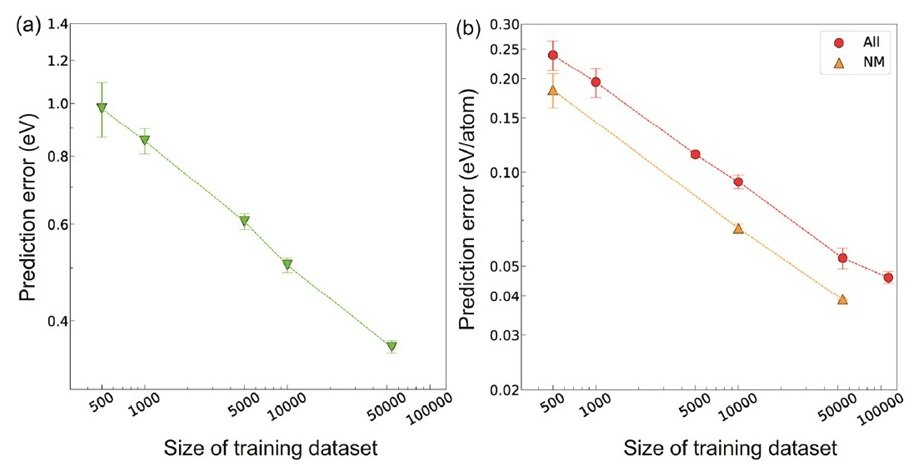The vertical and horizontal axes are log10 axes.

The prediction error decreased significantly as the data set size increased. The value of the prediction error at the maximum number of data was found to be comparable to the error of the value calculated by the first-principles calculation (DFT calculation) performed in conjunction with the experiment. In addition, the crystal graphs of metallic and nonmetallic inorganic materials are expected to be very different because the constituent elements and the types of bonds are different between them. Therefore, the model construction and prediction were performed separately for the metallic and nonmetallic materials, and the prediction error was significantly reduced when the two materials were separated under the same data size condition.

We then performed the prediction task with an insufficient number of data. The following figure shows a comparison of the prediction performance of CGCNN and TL-CGCNN for the bandgap (Eg) of non-metallic materials.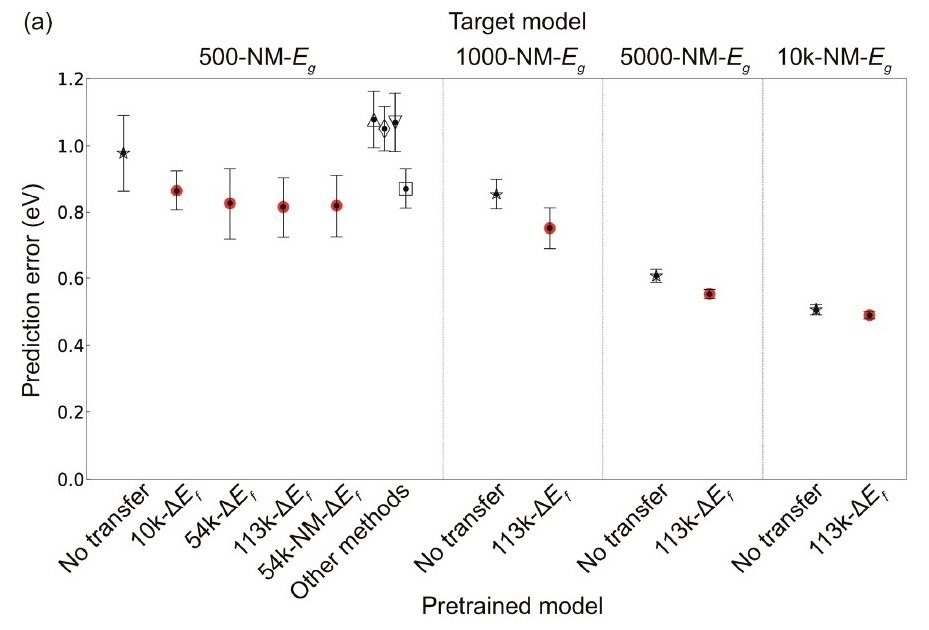TL-CGCNN has been pre-trained with data of each size. △: PLS, ▽: SVR, ◇: LASSO, ▢: RF

Compared to the CGCNN-only case, the prediction error was significantly lower when transition learning (TL) was performed. In addition, the prediction error of Eg decreased with increasing the amount of data pre-trained with the generative energy (ΔEf). 500-NM-Eg with the corresponding t-test at 1% significance level showed that the prediction error between CGCNN and TL-CGCNN pre-trained with 113k ΔEf data (113k-ΔEf TL-CGCNN), p = 6.9 × 10-5, confirming a clear improvement in prediction performance by TL-CGCNN.

Figure (a) below shows the comparison of the prediction performance of ΔEf for non-metallic materials by CGCNN and TL-CGCNN.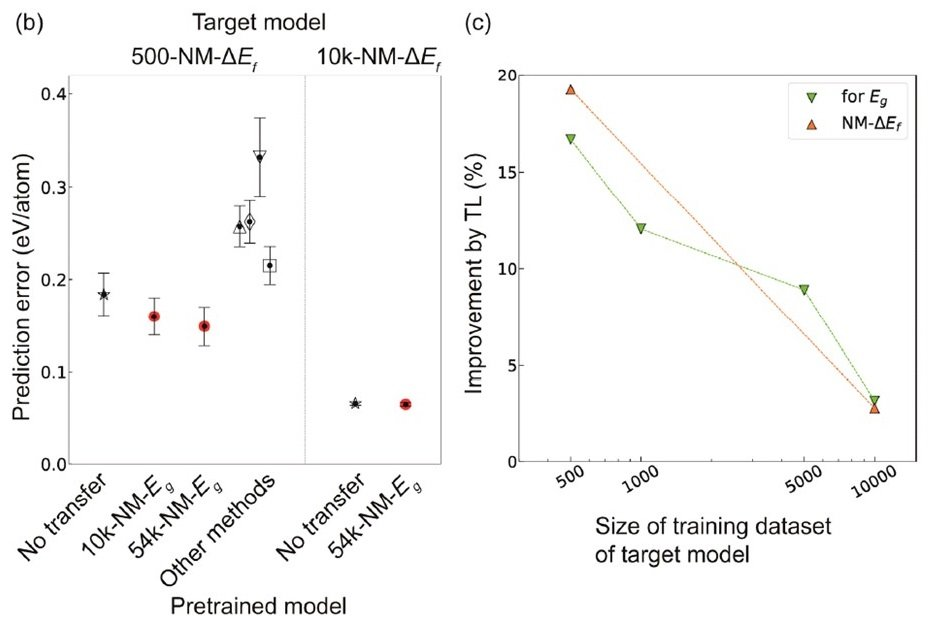TL-CGCNN is pre-trained with data of each size. △: PLS, ▽: SVR, ◇: LASSO, ▢: RF.

In TL-CGCNN, pre-training is performed using only non-metallic material data. Here too, a clear reduction in prediction error was observed with the use of TL-CGCNN.

Incidentally, no significant difference was found when ΔEf was predicted for data containing metallic substances after pre-training using only non-metallic substance data. This is probably because the constituent elements and bonding states of metallic and nonmetallic materials are very different, and the composition of the crystal graph is also very different. In other words, it may be better to use a different model for the property types of materials whose crystal graphs are expected to differ significantly.

The right figure (c) above shows the relationship between the amount of data for training the target model and the improvement rate of prediction accuracy when using TL. The smaller the amount of data in the target model, the more powerful the TL is.

### Application of TL-CGCNN to Prediction of Hard-to-Collect Property Data

Physical properties such as volume expansion rate ( KVRH ), dielectric constant ( εr ), and bandgap of quasiparticles (GW-Eg) are expensive to obtain by computational science, and the amount of data accumulated is very small compared to data such as Eg. CGCNN and TL-CGCNN (with low correlation to the above physical properties Pre-training with ΔEf and Eg ) to perform the task of predicting these properties, and a comparison of the prediction errors obtained is shown below.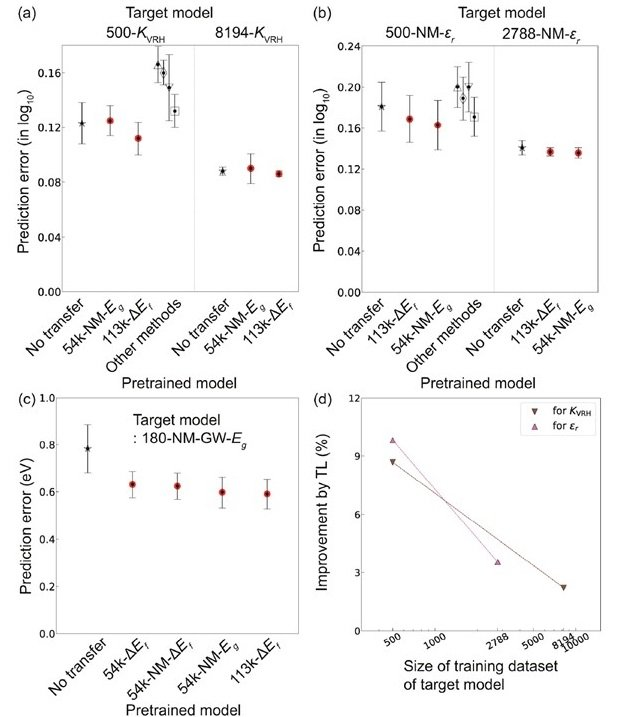Prediction errors for (a) volume expansion coefficient ( KVRH ), (b) dielectric constant ( εr ), and (c) quasiparticle bandgap (GW-Eg)

Performance improvement was observed for TL-CGCNN in all physical properties. In particular, the combined use of TL and CGCNN improved the prediction accuracy of GW-Eg for non-metallic materials by up to 24.5%. (Looking at the above figure, it looks like there is no significant difference, but the actual difference is much larger than it looks because the vertical axis is on a log10 scale.) In addition, the smaller the amount of training data for the target model, the higher the rate of improvement by TL became apparent.

Incidentally, the above results also show the results of similar tasks with well-known models such as PLS, SVR, RF, etc. Compared to the performance of CGCNN, only RF outperformed CGCNN for certain physical properties, even with a small number of data (500), but not nearly as well as TL-CGCNN. A widely used regression model such as RF performs well when combined with descriptors that are strongly correlated with the target variables, as in the present results. However, it is not easy to select a descriptor that is effective for each property value.

## summary

TL-CGCNN, which combines crystal graph descriptors and transition learning, is a powerful and flexible prediction model when only a small amount of data is available. The crystal graph descriptor shows excellent performance in transition learning because it can effectively grasp the characteristics of elements and crystal structures by pre-training on big data. From the above features, the TL-CGCNN model is expected to be useful for data collection of physical properties that are difficult to accumulate.

If you have any suggestions for improvement of the content of the article,